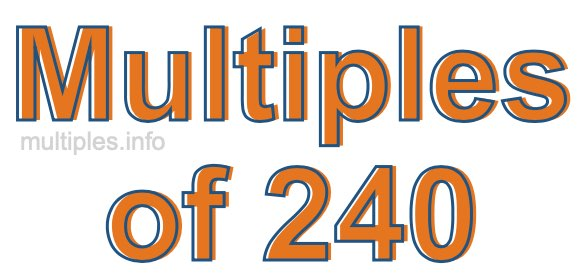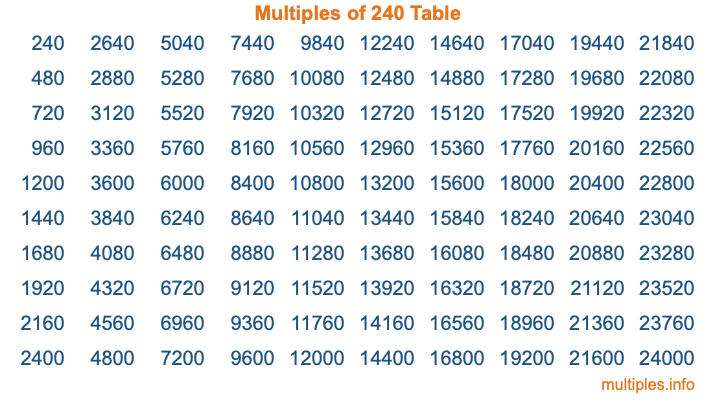Multiples of 240Welcome to the Multiples of 240 page. Here we will first teach you everything you will ever need to know about the multiples of 240, and then give you a study guide summary of everything we taught you to make sure you remember it all. Use this page to look up facts and learn information about the multiples of 240. This page will make you a multiples of two hundred forty expert!

Definition of Multiples of 240
Multiples of 240 are all the numbers that when divided by 240 equal an integer. Each of the multiples of 240 are called a multiple. A multiple of 240 is created by multiplying 240 by an integer.

Therefore, to create a list of multiples of 240, you start with 1 multiplied by 240, then 2 multiplied by 240, then 3 multiplied by 240, and so on for as long as you want. Thus, the list of the first five multiples of 240 is 240, 480, 720, 960, and 1200. To see a larger list of multiples of 240, see the printable image of Multiples of 240 further down on this page. We also have a category where you can choose any nth multiple of 240.

Multiples of 240 Checker
The Multiples of 240 Checker below checks to see if any number of your choice is a multiple of 240. In other words, it checks to see if there is any number (integer) that when multiplied by 240 will equal your number. To do that, we divide your number by 240. If the the quotient is an integer, then your number is a multiple of 240.

Is  a multiple of 240?

Least Common Multiple of 240 and ...
A Least Common Multiple (LCM) is the lowest multiple that two or more numbers have in common. This is also called the smallest common multiple or lowest common multiple and is useful to know when you are adding our subtracting fractions. Enter one or more numbers below (240 is already entered) to find the LCM.

Check out our LCM Calculator if you need more details about the Least Common Multiple or if you need the LCM for different numbers for adding and subtraction fractions.

nth Multiple of 240
As we stated above, 240 is the first multiple of 240, 480 is the second multiple of 240, 720 is the third multiple of 240, and so on. Enter a number below to find the nth multiple of 240.

th multiple of 240

Multiples of 240 vs Factors of 240
240 is a multiple of 240 and a factor of 240, but that is where the similarities end. All postive multiples of 240 are 240 or greater than 240. All positive factors of 240 are 240 or less than 240.

Below is the beginning list of multiples of 240 and the factors of 240 so you can compare:

Multiples of 240: 240, 480, 720, 960, 1200, etc.

Factors of 240: 1, 2, 3, 4, 5, 6, 8, 10, 12, 15, 16, 20, 24, 30, 40, 48, 60, 80, 120, 240

As you can see, the multiples of 240 are all the numbers that you can divide by 240 to get a whole number. The factors of 240, on the other hand, are all the whole numbers that you can multiply by another whole number to get 240.

It's also interesting to note that if a number (x) is a factor of 240, then 240 will also be a multiple of that number (x).

Multiples of 240 vs Divisors of 240
The divisors of 240 are all the integers that 240 can be divided by evenly. Below is a list of the divisors of 240.

Divisors of 240: 1, 2, 3, 4, 5, 6, 8, 10, 12, 15, 16, 20, 24, 30, 40, 48, 60, 80, 120, 240

The interesting thing to note here is that if you take any multiple of 240 and divide it by a divisor of 240, you will see that the quotient is an integer.

Multiples of 240 Table
Below is an image of the first 100 multiples of 240 in a table. The table is in chronological order, column by column. The first column has the first ten multiples of 240, the second column has the next ten multiples of 240, and so on.The Multiples of 240 Table is also referred to as the 240 Times Table or Times Table of 240. You are welcome to print out our table for your studies.

Negative Multiples of 240
Although not often discussed or needed in math, it is worth mentioning that you can make a list of negative multiples of 240 by multiplying 240 by -1, then by -2, then by -3, and so on, to get the following list of negative multiples of 240:

-240, -480, -720, -960, -1200, etc.

Multiples of 240 Summary
Below is a summary of important Multiples of 240 facts that we have discussed on this page. To retain the knowledge on this page, we recommend that you read through the summary and explain to yourself or a study partner why they hold true.

There are an infinite number of multiples of 240.

A multiple of 240 divided by 240 will equal a whole number.

240 divided by a factor of 240 equals a divisor of 240.

The nth multiple of 240 is n times 240.

The largest factor of 240 is equal to the first positive multiple of 240.

240 is a multiple of every factor of 240.

240 is a multiple of 240.

A multiple of 240 divided by a divisor of 240 equals an integer.

240 divided by a divisor of 240 equals a factor of 240.

Any integer times 240 will equal a multiple of 240.

Multiples of a Number
Here you can get the multiples of another number, all with the same attention to detail as we did for multiples of 240 on this page.

Multiples of
Multiples of 241
Did you find our page about multiples of two hundred forty educational? Do you want more knowledge? Check out the multiples of the next number on our list!